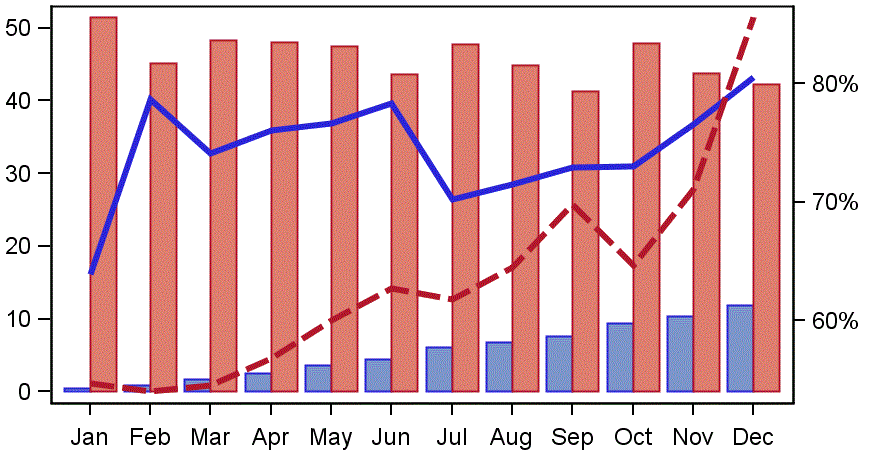## Setting bound on axis sgplot

I want my y2 axis to start at 0 (which I try to achieve by putting in min=0) but it is not working (see graph below). Here is my code

proc sgplot data=tariffprem_pol7 ;

vbarparm category=period response=count / group=Business ;

series x=period y=aptp / group=Business lineattrs=(thickness=3) y2axis ;

y2axis min=0 offsetmin=0;

yaxis min=0 offsetmin=0;

yaxis label = "Policy Count";

y2axis label = "AP/TP";

xaxis label = "Month (2015 Year)";

run;Any ideas where I'm going wrong?

1 ACCEPTED SOLUTION

Accepted Solutions

## Re: Setting bound on axis sgplot

Hi @brophymj,

I think, the reason is that you have two Y2AXIS statements. The options of the second one will override those of the first. So, please add the option label= "AP/TP" to the first Y2AXIS statement and delete the second Y2AXIS statement (and fix the similar issue with the two YAXIS statements).

5 REPLIES 5

## Re: Setting bound on axis sgplot

The documentation of the Y2AXIS statement says: "[The MIN=] option does not determine the minimum axis tick value displayed. The THRESHOLDMIN= value is used to determine the minimum tick value." But the VALUES= option, which Reeza alluded to, gives you more control over the values displayed. Depending on your data, VALUES=(0 to 80 by 10) or VALUES=(0 to 0.8 by 0.1) should work.

## Re: Setting bound on axis sgplot

Hi

I tried putting in the values but it didn't work. here is  my code:

ods graphics / reset width=5in height=3in imagename='ROI Fleet APTP for 2015';

Title "ROI Fleet Earned APTP for 2015 (Split NB & Ren)";

proc sgplot data=tariffprem_pol7 ;

vbarparm category=period response=count / group=Business ;

series x=period y=aptp / group=Business lineattrs=(thickness=3) y2axis ;

y2axis min=0 values=(0 to 0.8 by 0.1) offsetmin=0;

yaxis min=0 offsetmin=0;

yaxis label = "Policy Count";

y2axis label = "AP/TP";

xaxis label = "Month (2015 Year)";

run;

## Re: Setting bound on axis sgplot

Hi @brophymj,

I think, the reason is that you have two Y2AXIS statements. The options of the second one will override those of the first. So, please add the option label= "AP/TP" to the first Y2AXIS statement and delete the second Y2AXIS statement (and fix the similar issue with the two YAXIS statements).

## Re: Setting bound on axis sgplot

Thanks, that worked
Discussion stats
• 5 replies
• 2452 views
• 0 likes
• 3 in conversation